## She Loves Data: R Workshop Day 1 – ListLists are straightforward type of data structure, by using list().

Syntax: list(vector, val2, …)
Lists:
– Store an ordered collections of objects.
– It can contain different data types, works like a container without restriction.

`#Define a list with vector, floating number and function, sin.list <- list(c(2,3,5), 31.7, sin)`

The output on my console shows,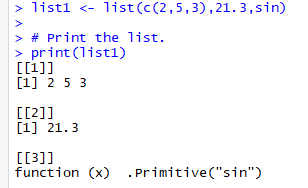Three different elements list on the console.
Another example of using list was in my previous blog on Matrix, where I define the dimnames using list in the example.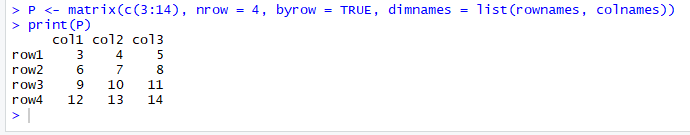The rownames and colnames are vectors which contain the names of the rows and columns.

I do not have many example using list() especially on how to access the elements of the list. However, I believe it should be able to retrieve the elements similarly to other programming languages by using the indexes and square brackets. I will give an update on that later.

## She Loves Data: R Workshop Day 1 – Data FrameIt is an important data structure in R.

Syntax: data.frame() everything will be declared within the parenthesis.
Data Frames:
– Generated by combining multiple vectors
– It can be created by using external files when importing the data into R.

I am not sure how to share what I learned about data frame in just one blog entry. It works slightly different than matrices, where data frame can contain different modes of data. See example below:

`#Create the data frame. emp.data <- data.frame(   emp_id = c(1:5),    emp_name = c("Rick","Dan","Michelle","Ryan","Gary"),   salary = c(623.3,515.2,611.0,729.0,843.25),  start_date = as.Date(c("2012-01-01", "2013-09-23", "2014-11-15", "2014-05-11","2015-03-27")) )#Print the data frame. print(emp.data) `

A data frame is created called emp.data which contains of number for emp_id, characters for emp_name, floating points for salary and date for start working date. The output of the data frame on the console when I print(emp.data) is as below: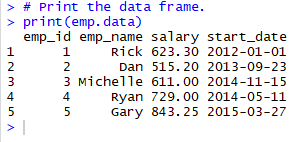In data frame, the column names are taken from the variable names of the vectors.

Data frame has several built-in R functions which are quite useful. Follow the examples below:

str(emp.data)
– When I execute the above code, the console shows:
‘data.frame’: 5 obs. of 4 variables:
\$ emp_id : int 1 2 3 4 5
\$ emp_name : Factor w/ 5 levels “Dan”,”Gary”,”Michelle”,..: 4 1 3 5 2
\$ salary : num 623 515 611 729 843
\$ start_date: Date, format: “2012-01-01” “2013-09-23” “2014-11-15” “2014-05-11” …

Do you know why it is 5 objects? Yes, 4 vectors and a data frame.

View(emp.data)
– View the data in tabular format.
– Navigate to the top left box in the RStudio, I see another tab named with empdata displayed.
– Use it often to check or view data.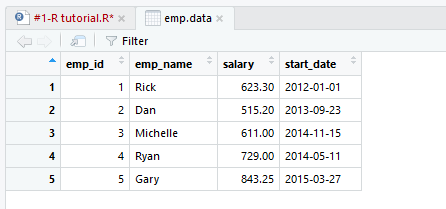Cool, right?

Next cool things we can do with data frame is using the summary(emp.data).
– Print out the summary and it shows the min, max, median, mean, 1st quarter and 3rd Quarter. In some statistics analysis, this is very useful piece of information.
– How to do extract just min, median and max values from the summary()?

What if I want to extract specific columns from the data frame? How does it can be done? Below codes explain and the output on the console. I can access to the columns in the data frame by using “\$” symbol.

`#Extract Specific columns. result <- data.frame(emp.data\$emp_name,emp.data\$salary) print(result)`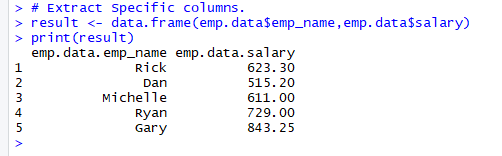Accessing the data frame.
– Extract information of a specific rows and columns.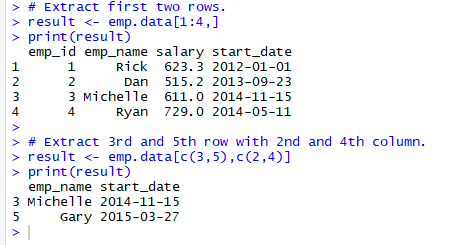– Extract using head() and tail().
In a larger data frame, it is quite useful function to extract top 6 records and last 6 records. The example from the workshop is not large enough to see the different, so let try head(mtcars) and tail(mtcars).

mtcars is built-in data frame in RStudio.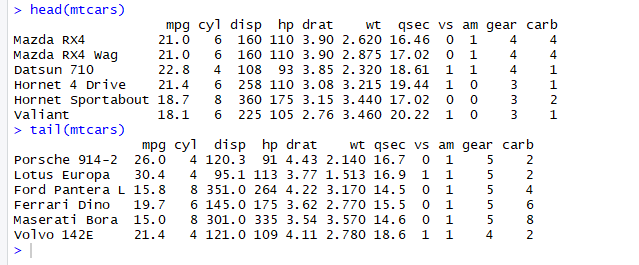To add another “column”, it can be done directly with codes below:
emp.data\$dept < – c(“IT”,”Operations”,”IT”,”HR”,”Finance”).

Then, map it to a variable to print out on the console using the following codes,

`#Add the "dept" column. emp.data\$dept <- c("IT","Operations","IT","HR","Finance") v <- emp.data print(v)`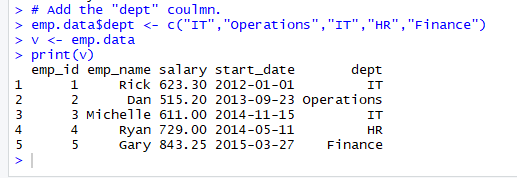The key is using “\$“, the same key I used to extract or access data from emp.data data frame.

I will share more on data frames when I come across interesting codes.  Stay tuned. Thank you.

## She Loves Data: R Workshop Day 1 – MatrixI continue with the next data structure in R language, let have a quick zoom into matrices with example given by the instructor as below using the matrix syntax:

Syntax : matrix(data, nrow, ncol, byrow, dimnames)

Matrices:
– Elements are arranged sequentially by row.
– It starts with row, then column.
data can be a form of vector.
nrow or ncol means desired number of rows or columns.
byrow is a logical value, TRUE or FALSE. By default, the matrix is filled by columns, otherwise the matrix is filled by rows.

Let me share the examples from the workshop.

`#matrices #Elements are arranged sequentially by row. M <- matrix(c(3:15), nrow = 4, byrow = TRUE) print(M) #Elements are arranged sequentially by column. N <- matrix(c(3:14), nrow = 4, byrow = FALSE) print(N)`

My output on the console,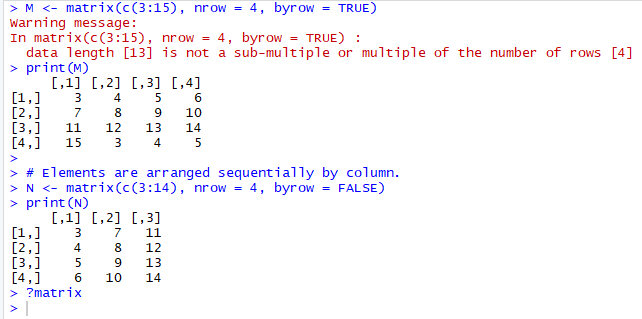Notice the above warning message. It is because the length of the vector c ranging from number 3 to 15, inclusive, is only 13 and I wanted to create a 4 rows matrix (m) by setting nrow = 4, byrow = TRUE.

However, if I changed byrow = FALSE, the 13 elements are arranged by column and returned the matrix (n) without warning message.

Rename rows and columns in matrix
It allows to define the row and column names using dimnames.

`#Define the column and row names. rownames = c("row1", "row2", "row3", "row4") colnames = c("col1", "col2", "col3") P <- matrix(c(3:14), nrow = 4, byrow = TRUE, dimnames = list(rownames, colnames)) print(P)`Rename the row and column names gives us a better picture of the matrix.

Before I move on, do you notice the above codes contains vector, matrix and list? Amazing, right?

Does Python or Scala able to do so? Please share with me if you know it.

Access elements in matrix
I can access the element in the matrix using index, which begin with 1. Some example using the matrix (p) to find some values.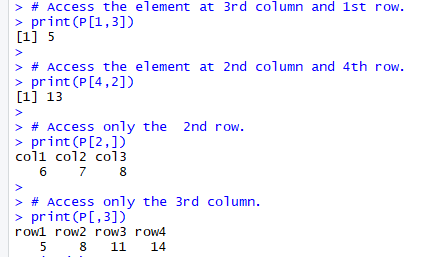Matrix of the same data type and number of elements within a matrix, can perform addition of both matrix together or subtraction values between the matrices.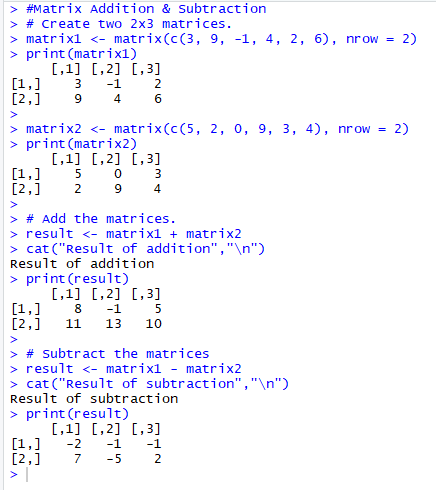Pretty cool right, working around with matrices. I am going to share two more data structures, the list and the data frame in my next blogs.

## She Loves Data: R Workshop Day 1 – VectorsAfter a short break over the weekend, I began my learning again. Let continue exploring the data structure in R language, the vectors. The basic ones to play around and get familiar with codes.

Syntax: c(val1, val3, val3, …)

Vectors
– Simplest, basic data structure in R.
– Contains same type of data.

`#Vector declaration#vector containing three numeric values 2, 3 and 5. c(2, 3, 5)#vector contains 1 to 10. First value before colon (:) is start number, and value after the colon is end number.c(1:10)#vector of logical values.  c(TRUE, FALSE, TRUE, FALSE, FALSE)  #vector of character values.c("aa", "bb", "cc", "dd", "ee") #Check the length of the vector and it returns 5.length(c("aa", "bb", "cc", "dd", "ee")) #check the length of a value, "aa" in the vector. It returns 2.nchar( c("aa")) #check the data type,n = c("aa", "bb", "cc", "dd", "ee")  class(n) "character"typeof(n) "character" #Full codes:   c("aa", "bb", "cc", "dd", "ee")     "aa" "bb" "cc" "dd" "ee"   length(c("aa", "bb", "cc", "dd", "ee"))    5   nchar( c("aa"))    2   n = c("aa", "bb", "cc", "dd", "ee")    class(n)    "character"   typeof(n)    "character"  #Combining vectors. It converts the numeric into string.   n = c(2, 3, 5)    s = c("aa", "bb", "cc", "dd", "ee")    c(n, s)    "2"  "3"  "5"  "aa" "bb" "cc" "dd" "ee"   `

I learned from the recent R workshop organized by Sparkline, the vectors can be used in many different situations. I will try to point it out whenever I share the codes in my next blogs. Let us move on to the next data structure.

## Python: Pig Latin

• If word starts with a vowel, add ‘ay’ to end.
• If word does not start with a vowel, put first letter at the end, then add ‘ay’
• Example:
• word > ordway
• apple > appleay

To further understand and practise the functions in Python, the tutor shared one of the case studies in the online web learning. The case study detail is as above and the workaround is taking the first character and check if it matches the condition.

If yes, then appends the ‘ay’ to the end.
If no, then takes the 2nd character onward, appends the 1st character and follows by appending ‘ay’ to the end.

Sample code as below screenshot: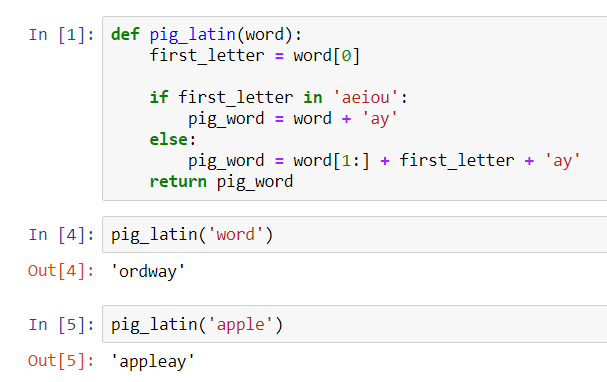## Day 18: Functions in Python

Continuous from the introduction of functions and methods in Python in my previous blog entry. I added a few more examples to understand functions better. In the next examples, I extracted it from DataCamp and Udemy’s online web learning courses.

Built-in Functions
Most of us familiar with len(), str(), format() or print() which I used as examples in previous blog entries. These are built-in Functions from Python which we used to get the length of the string, converting a value to a string and format the value to print out a line accordingly.

Hope, till here, it is simple to go ahead with an example of str() to recap. See below for code snippet and the output from the Jupyter Notebook.

```x = str(10)
print(x)
print(type(x))
```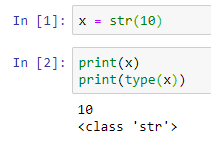It prints out the value 10 as an ‘str’ (string) class. Do you still remember, if we want to print a number, we convert the number to string data type and concatenate with other strings of words (if any) to print out the whole statement? The str() converts number to string. Otherwise, it returns an int data type and it cannot be concatenated with strings.

Now, move on to defining our functions with examples and explanations below. Still remember the keywords for defining a function?

• Keyword def marks the start of function’s header.
• Function name, abc, is uniquely named.
• Parameters (arguments) which will pass values to the function. It is optional. In the example, a and b are the values pass to the function.
Colon (:) marks the end of the function’s header.
• Indentation after the newline.
• Docstring, an optional documentation to describe what the function does, usually helpful to developers.
• Execution statements.
• Return statement to return a value from the function. It is optional.

## Defining a function without a parameter

Let begin to write a simple user defined function with the code snippet below based on the function’s syntax I mentioned above. We choose to leave the parameter as an option, so we do not define it.

```def square():
new_value = 2 ** 2
print(new_value)
print(type(new_value))

square()
```

After writing the function block of codes, we need to call our function. The above function does not do or return anything on the screen unless we do a call or we invoke the function. That is why the next line of code is required:
square()

Upon execution, it prints the value on the screen accordingly. It does not require any input of arguments in this code. And the value is fixated to use,
new_value = 2 ** 2

every time it is being called. it returns the same output.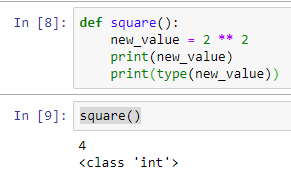## Defining a function with a parameter and provide a default value of a parameter.

How about now, we put in the parameter of our function? How to code it, example below:

```def sayhello(name):
print('hello ' + name)
```

When I call the function sayhello() with an argument, syntax like this,
sayhello(‘Li Yen’)

the output on the screen shows,
hello Li Yen

Up to here, probably, you want to know why sometimes I use parameters and arguments. The DataCamp’s tutorials on Python Data Science Toolbox, gave a good example to example this. The parameter is used when defining a function and we call a function, we pass an argument into the function to be executed. I hope I can make it clear enough and try to distinguish these two jargon.

What if I did not input a value (argument) to the function? The above function expects a value and if we execute it without a value, Python throws an error.

While the parameter defines to accept a value (argument) from the user, it can be set with a default argument by giving it a variable and a value such as name = ‘User’. The variable, name has a value of ‘User’. This is how the code looks like,

```def sayhello(name='User'):
print('hello ' + name)
```

So, when we call the same function again without passing argument, syntax like this,
sayhello()

the output on the screen shows,
hello User

Another example below, showing the combination of using the default value, yet I’m passing my name into the function. It overwrites the value of ‘User’. Cool!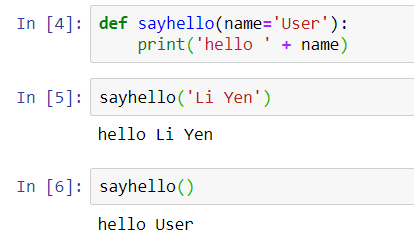## Multiple Function Parameters

Accept more than 1 parameter and when we call a function, number of arguments same as number of parameters. The values are assigned in sequence of the parameters.

Call function: # of arguments = # of parameters. Below is the examples of multiple parameters:

```def raise_to_power(value1, value2):
"""Raise value1 to the power of value2."""
new_value = value1 ** value2
return new_value

result = raise_to_power(2, 3)
print(result)
```
```def square(value1, value2):
new_value = value1 ** value2
return new_value

square(10,3)
```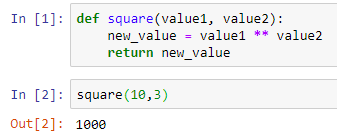Is it straight forward enough with the above example code and output? When the square(10,3) is called, the value of 10 passes to variable, value1 and the value of 3 passes to variable, value2, then the variable new_value holds the value of the computation of value1 ** value2. In syntax format, it looks like this,

```new_value = 10 ** 3
```

To call the square() function, declare a variable result and print the output of result:

```result = square(10,3)
print(result)
```

## Return statement

Return a Boolean from a function
We can return a Boolean from a function, just like other data types too. The example below, I’m returning a Boolean’s value.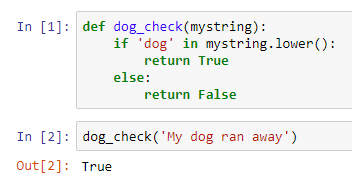If we check the condition as below,

```'dog' in 'My dog ran away'
```

It returns True.

So, in that example, the tutor said, we can simplify the codes by removing the if statement to achieve the same result. It can be done in one line.

```def dog_check(mystring):
return 'dog' in mystring.lower()
```

## Return multiple values

As mentioned in my summary post, the return statement can return more than one value. When I learned it through DataCamp, this can be happened when a function returns a tuple. We covered what tuple is. It is a collection of data, just like a list of parentheses. It is immutable, which means data cannot be changed.
Tuple (‘a’, ‘b’, ‘c’, ‘d’)

Below is the screenshot of the sample code: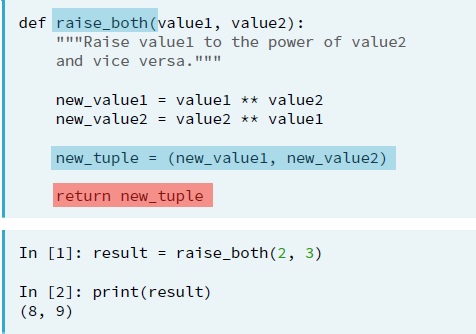Another sample code from DataCamp, an expand from the above square() function to return 2 values in a tuple, unpack the tuple and print out a value using index.

```def square(value1, value2):
new_value1 = value1 ** value2
new_value2 = value2 ** value1

new_tuple = (new_value1, new_value2)
return new_tuple

result = square(10,3)
print(result)
print(result) #print(square(10,3))
print(result) #print(square(10,3))
```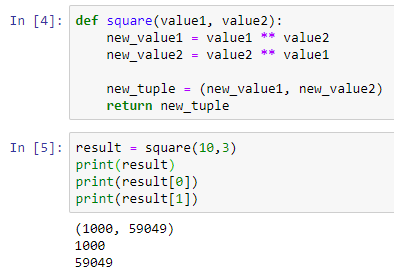The above shows a combination of using functions with multiple parameters, returning the multiple values using a tuple and print out the result by unpacking the tuple and print out the result of new_value1 and new_value2 by its index.

Summary of the day:

• Define function without parameter.
• Define a function with a parameter.
• Use the default argument in the parameter.
• Use the return statement with a single value.
• Use the return statement with tuple for multiple values.
• Unpack tuple and using index slicing to print the value of a tuple.

## Day 18: Methods and Functions in Python

I did some revision before I continued with the new chapter of my learning in Udemy’s Complete Python Bootcamp, From zero to hero. I tried to look for a simpler explanation of the differences between methods and functions in Python.

Trying to put it in a dummy way to explain sometimes, it requires a good example, just like this one I found in Quora.com, written by Sakina Mirza.

A user-defined function.
A function is a block of code to carry out a task and it calls by its name. All functions may have zero or many arguments. The arguments are passed explicitly (directly). On the exit of the function, it may or may not return value or values.

Python allows us to define our own function calls add with a and b as the arguments, just like an example below:

```def add(a,b):
return a + b
```

Main things to remember:

• Keyword def marks the start of function’s header.
• Function name, abc, is uniquely named.
• Parameters (arguments) which will pass values to the function. It is optional. In the example, a and b are the values pass to the function.
• Colon (:) marks the end of the function’s header.
• Indentation after the newline.
• Docstring, an optional documentation to describe what the function does, usually helpful to developers.
• Execution statements.
• Return statement to return a value from the function. It is optional.

How to call the above function in Python?
We can call it from another function, program or the Python prompt. To call a function, simply type the function name with the parameters if specified.

In our example, it types as,

```abc(7, -4)
```

The return statement is used to exit a function and go back to the point where it was called. If a function does not have a return statement, then the function will return the None object. Function ends here and usually, it could be a printout statement to indicate end of a function.

Our return value is 3 for the above.

Other examples of functions are the common ones we used before this, such as len(), str(), format() or even print(). These are the built-in functions in Python and they return values or output when we call it. So, 2 types of functions to be remembered in Python, built-in functions and user defined functions.

Python Methods.
It is like a function, except it is attached to an object (dependent). A method is implicitly (indirectly) passed to the object for which it is invoked. It may or may not return a value or values. The method is accessible to data that is contained within the class.

This means we call a method (which is a function belongs) on (to) an object and it possibly makes changes to that object. Let take an example below:

```class vehicle:
def __init__(self, color):
self.color = color
def start(self):
print("Starting engine")
def showcolor(self):
print(f"I am {self.color}")
```

Main things to remember:

• Keyword class marks the start of the header.
• Class name, vehicle, is uniquely named.
• Colon (:) marks the end of the function’s header.
• Indentation after the newline.

How to call a method?
We create an object called, car and we can use the class named, vehicle. Noticed, the example used,
__init__() function

It is an built-in function in Python. All classes have a function called __init__() which is executed when the class is being initiated. Use it to assign values to object properties or other operations that are necessary to do when the object is being created.

So, that explained the first part of the codes above when we assign ‘black’ to the object ‘car’.

```car = vehicle('black')
car.start()
car.showcolor()
```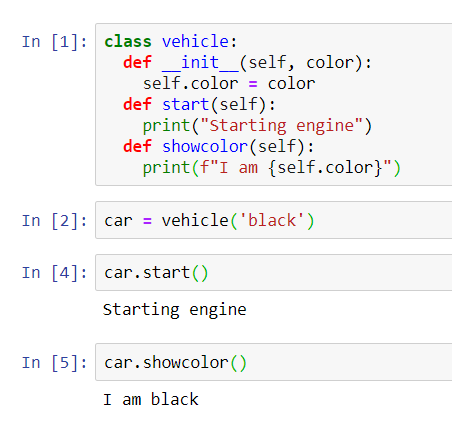When we called the functions, start() and showcolor() from the object, car, the print out the statements as shown above. I will share a bit more examples I extracted from the online web learning course in my next blog.

Summary of the day:

• User-defined function and built-in function.
• Method, class and object.